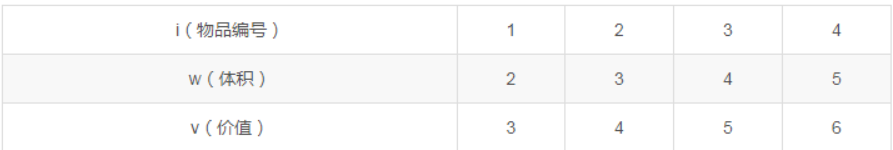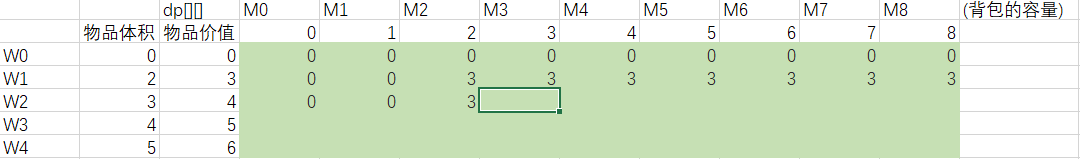# 动态规划解0-1背包问题

• 最优子结构性质
• 重叠子问题

## 0-1背包问题

``````有n个物品，它们有各自的体积和价值，现有给定容量的背包，如何让背包里装入的物品具有最大的价值总和？
``````## 背包问题抽象化

W0-W4是物品编号，M0-M8是背包的容量，表中绿色区域表示dp值，即该背包容量下，前n个物品可装入的最大价值。``````int W,C;//w是物品重量，C是物品价值,测试数量不能超过30
int main()
{
int,m,n;//m为背包容量，n为物品数量
scanf("%d%d",&m&n);
for(int i=0;i<n;i++){
scanf("%d%d",&w[i]&c[i]);
}
for(int i = 1;i<=n;i++){
for(int j =1;j<=m;j++){
if(j>w[i]){
dp[i][j]=dp[i-1][j];
}else{
dp[i][j]=max(dp[i-1][j],dp[i-1][j-w[i]]+c[i]);
}
}
}
}
``````

## 代码优化

``````#include<stdio.h>
int main() {
//动态规划解0-1背包问题
//递推公式：dp[j]=dp[j-w[i]]+v[i]
int m, n;//m为背包容量，n为物品数量,n小于20；
int w, v,dp;//w[i]为第i件物品的重量，v[i]是第i件物品的价值,dp[]为递推数组
scanf("%d %d", &m, &n); //m为背包容量，n为物品数量,n小于20；
for (int j = 0; j<= m; j++) {
dp[j] = 0; //dp置零
}
for (int i = 1; i <= n; i++) {
scanf("%d %d", &w[i], &v[i]);
}
for (int i = 1; i <= n; i++) {
for (int j = m; j >= 0; j--) {
if (j >= w[i]) {
dp[j] = dp[j - w[i]] + v[i];
}
}
}
printf("%d", dp[m]);
return 0;
}
``````